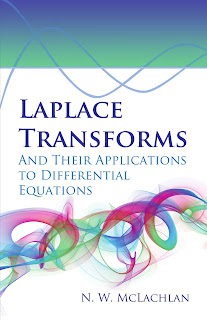DISCRETE MATHEMATICS

# Laplace Transforms and Their Applications to Differential Equations PDF Free DownloadClassic graduate-level exposition covers theory and applications to ordinary and partial differential equations. Includes derivation of Laplace transforms of various functions, Laplace transform for a finite interval, and more. 1948 edition. This introduction to modern operational calculus offers a classic exposition of Laplace transform theory and its application to the solution of ordinary and partial differential equations. The treatment is addressed to graduate students in engineering, physics, and applied mathematics and may be used as a primary text or supplementary reading. Chief topics include the theorems or rules of the operational calculus, evaluation of integrals and establishment of mathematical relationships, derivation of Laplace transforms of various functions, the Laplace transform for a finite interval, and other subjects. Many problems and illustrative examples appear throughout the book, which is further augmented by helpful Appendixes.